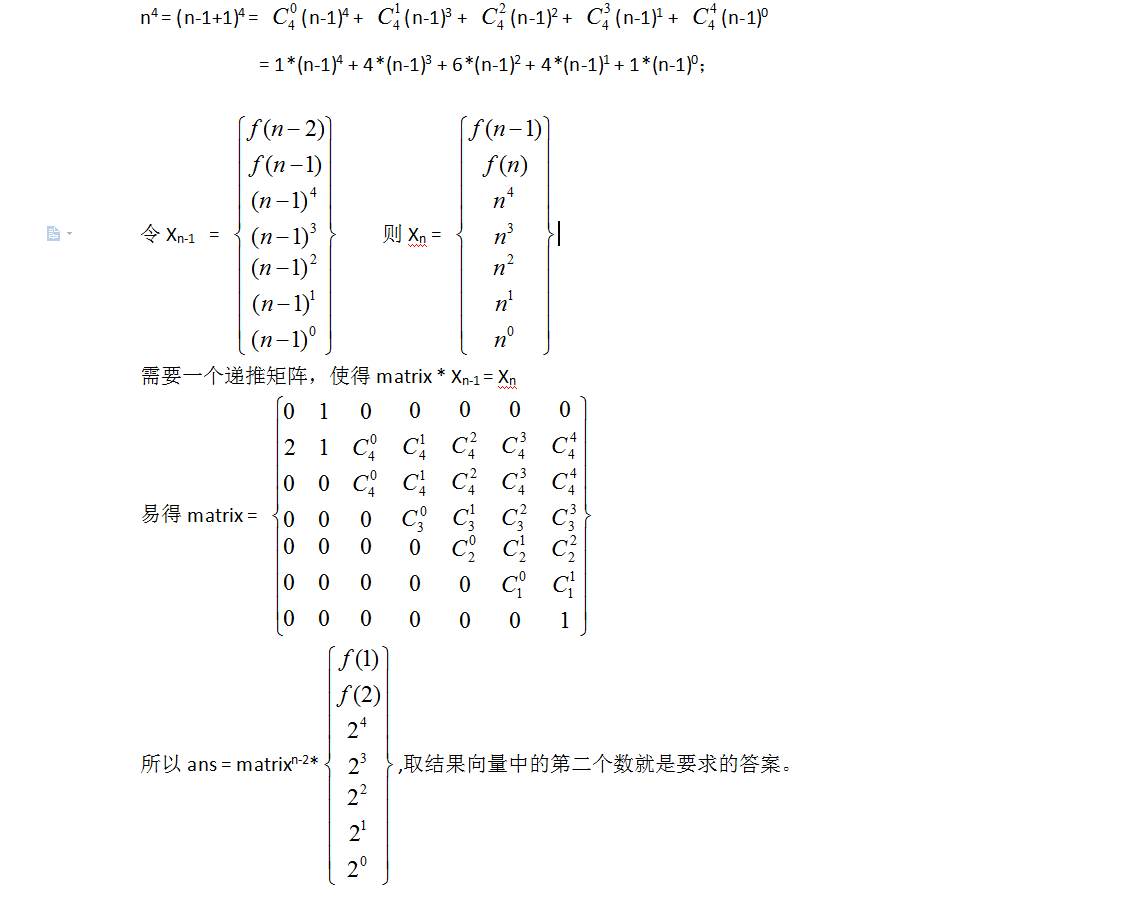# Recursive sequence（快速矩阵幂模板）

### 该类题型适合大数，递推式及一个状态到另一个状态的规律

Problem Description

Farmer John likes to play mathematics games with his N cows. Recently, they are attracted by recursive sequences. In each turn, the cows would stand in a line, while John writes two positive numbers a and b on a blackboard. And then, the cows would say their identity number one by one. The first cow says the first number a and the second says the second number b. After that, the i-th cow says the sum of twice the (i-2)-th number, the (i-1)-th number, and i4. Now, you need to write a program to calculate the number of the N-th cow in order to check if John’s cows can make it right.

Input

The first line of input contains an integer t, the number of test cases. t test cases follow.
Each case contains only one line with three numbers N, a and b where N,a,b < 231 as described above.

Output

For each test case, output the number of the N-th cow. This number might be very large, so you need to output it modulo 2147493647.

Sample Input

2

3 1 2

4 1 10

Sample Output

85

369

Hint

In the first case, the third number is 85 = 2*1十2十3^4. In the second case, the third number is 93 = 2*1十1*10十3^4 and the fourth number is 369 = 2 * 10 十 93 十 4^4.

Source

2016ACM/ICPC亚洲区沈阳站-重现赛（感谢东北大学）

### 注意：该处必须要凑的矩阵为n*n的，这样才能够自己相乘！！！n^4的拆分很巧妙### 代码：

 //记住模板，关键还是在于找规律
#include "bits/stdc++.h"
#define rep(i,j,k) for(int i=j;i<=k;i++)
const int MOD = 1e5+7;
typedef long long ll;
using namespace std;
const int N =7;
ll mod = 2147493647;//必须是long long

struct mat
{
ll a[N][N];
};

mat mul_mat(mat &a,mat &b)
{
mat res;
memset(res.a,0,sizeof(res.a));
rep(i,0,6)
rep(j,0,6)
rep(k,0,6)
{
res.a[i][j]=(res.a[i][j]+a.a[i][k]*b.a[k][j])%mod;
}//就是这个位置了，之前用res.a[i][j]+a.a[i][k]*b.a[k][j]%mod;
//一直ac不了，还是要打括号保险
return res;
}
mat pow_mat(ll n,mat &a)
{
mat res;
memset(res.a,0,sizeof(res.a));
for(int i=0;i<=6;i++)
res.a[i][i]=1;
while(n)
{
if(n&1) res=mul_mat(res,a);
a=mul_mat(a,a);
n>>=1;
}
return res;
}
int main()
{
int n;
cin>>n;
while(n--)
{
int a,b,m;
cin>>m>>a>>b;
int c[]={a,b,16,8,4,2,1};
if(m==1)
cout<<a<<endl;
else if(m==2)
cout<<a<<endl;
else
{
mat res=
{
0,1,0,0,0,0,0,
2,1,1,4,6,4,1,
0,0,1,4,6,4,1,
0,0,0,1,3,3,1,
0,0,0,0,1,2,1,
0,0,0,0,0,1,1,
0,0,0,0,0,0,1,
};
mat cur=pow_mat(m-2,res);
ll sum=0;
for(int i=0;i<=6;i++)
{
sum=((sum+cur.a[i]*c[i])%mod);
}
cout<<sum<<endl;
}
}

return 0;
}

11-07212
08-013810-09402
04-06162
08-1087
09-1217
09-12139
01-0369
09-11
09-29